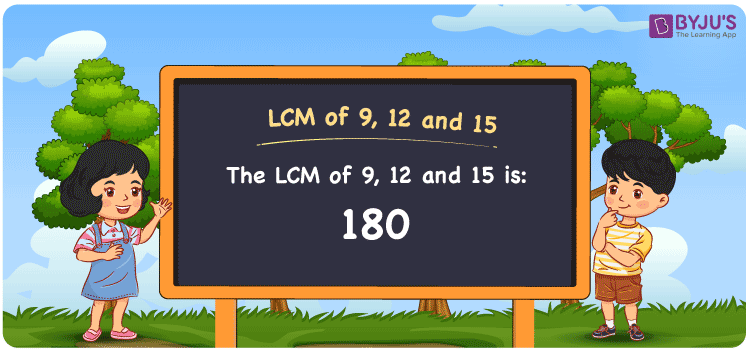# LCM of 9 12 and 15

LCM of 9, 12 and 15 is 180. LCM stands for least common multiple. It is also called LCD. Subject matter experts crafted the article Least Common Multiple (LCM) with the intention to provide students with accurate study material to obtain a thorough knowledge of the concept. Referring to this article while solving the problems based on the LCM helps students to tackle any type of problem in exams effortlessly. In this article, let us grasp the simple technique to find the least common multiple of 9, 12 and 15 in an efficient way.

## What is LCM of 9, 12 and 15?

The Least Common Multiple of 9, 12 and 15 is 180.## How to Find LCM of 9, 12 and 15?

LCM of 9, 12 and 15 can be determined using three methods

• Prime Factorisation
• Division method
• Listing the multiples

### LCM of 9, 12 and 15 Using Prime Factorisation Method

In the prime factorisation method, the given natural numbers are expressed as the product of prime factors. The least common multiple will be the product of all prime factors with the highest degree.

9 = 3 × 3

12 = 2 × 2 × 3

15 = 3 × 5

LCM (9, 12, 15) = 2 × 2 × 3 × 3 × 5 = 180

### LCM of 9, 12 and 15 Using Division Method

In the division method, to calculate the least common multiple of 9, 12 and 15, we divide the numbers 9, 12 and 15 by their prime factors until we get the result as one in the complete row. The product of these divisors depicts the least common multiple of 9, 12 and 15.

 2 9 12 15 2 9 6 15 3 9 3 15 3 3 1 5 5 1 1 5 x 1 1 1

No further division can be done.

Hence, LCM (9, 12, 15) = 2 × 2 × 3 × 3 × 5 = 180

### LCM of 9, 12 and 15 Using Listing the Multiples

In this method, we list the multiples of 9, 12 and 15 to calculate the least common multiple among them. Let us glance at the multiples of 9, 12 and 15 given below.

Multiples of 9: 9, 18, 27, 36, 45, 54, 63, 72, 81, 90, ……….., 162, 171, 180, ……

Multiples of 12: 12, 24, 36, 48, 60, 72, 84, 96, 108, 120, ………., 156, 168, 180, …..

Multiples of 15: 15, 30, 45, 60, 75, 90, 105, 120, ………., 150, 165, 180, …….

LCM (9, 12, 15) = 180

## Video Lesson on Applications of LCM## Solved Example

Question: What is the smallest number that is divisible by 9, 12, 15 exactly?

Solution: The smallest number that is divisible by 9, 12 and15 exactly is their LCM. We know that the LCM of 9, 12 and 15 is 180. Hence the smallest number that is divisible by 9, 12, 15 exactly is 180.

## Frequently Asked Questions on LCM of 9, 12 and 15

### What is the LCM of 9, 12 and 15?

The LCM of 9, 12 and 15 is 180.

### Is the LCM of 9, 12 and 15 the same as the HCF of 9, 12 and 15?

No. The LCM of 9, 12 and 15 is 180 and the HCF of 9, 12 and 15 is 3.

### 190 is the LCM of 9, 12 and 15. True or False.

False. The LCM of 9, 12 and 15 is 180.

### Write the methods used to find the LCM of 9, 12 and 15.

The methods used to find the LCM of 9, 12 and 15 are

Prime Factorisation

Division Method

Listing the Multiples

### How to find the LCM of 9, 12 and 15 using the prime factorisation method?

In this method, we express the numbers as the product of prime factors

9 = 3 × 3

12 = 2 × 2 × 3

15 = 3 × 5

LCM (9, 12, 15) = 2 × 2 × 3 × 3 × 5 = 180